## Example Questions

### Example Question #1 : Statistics

The medians of the following two sets of numbers are equal, and
the sets are arranged in ascending order
{1, 4, x, 8} and {2, 5, y, 9}. What is y – x?

3

2

–1

–2

0

–1

Explanation:

Explanation: Recall that the median of an even-numbered set of numbers is the arithmetic mean of the pair of middle terms. Thus (4 + x)/2 = median of the first set and (5 + y)/2 = median of the second set. Since both medians are equal, we can set the equations equal to eachother. (4 + x)/2 = (5 + y)/2. Multiply both sides by 2 and we get 4 + x = 5 + y. We also know that 4 < x < 8 and 5 < y < 9, since the sets are arranged in ascending order. This narrows our options for x and y down significantly. Plugging in various values will eventually get you to x = 7 and y = 6, since 7 + 4 = 11 and 5 + 6 = 11, and thus the median in both cases would be 5.5. Thus, y – x = –1.

### Example Question #1 : Statistics

What is the median in the following set of numbers?

16, 19, 16, 7, 2, 20, 9, 5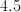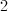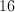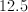Explanation:

16, 19, 16, 7, 2, 20, 9, 5

Order the numbers from smallest to largest.

2,5,7,9,16,16,19,20

The median is the number in the middle.

In this case, there is a 9 and 16 in the middle.

When that happens, take the average of the two numbers.

### Example Question #1 : Statistics

Find the median: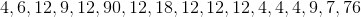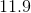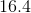Explanation:

To find the median, arrange the numbers from smallest to largest:

4,4,4,4,6,7,9,9,12,12,12,12,12,12,18,76,90

There are 17 numbers in total.  Since 17 is an odd number, the median will be the middle number of the set.  In this case, it is the 9th number, which is 12.

### Example Question #1 : Statistics

There are 3,500 people in group A and 5,000 people in group B:

 Car Type % in Group A Who Own % in Group B Who Own Motorbike 4 9 Sedan 35 25 Minivan 22 15 Van 9 12 Coupe 3 6

What is the median of the number of people in group B who own either a minivan, van, or coupe?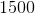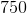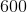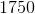Explanation:

Treat the percentages as a list, as we are including every demographic from the 3 vehicle types mentioned. If we do each 0.06(5000), 0.12(5000), and 0.15(5000) we note from observation that the median, or middle value, would have to be the 12% row since the sample size does not change. The question asks for EITHER of the 3 categories, so we can ignore the other two.

0.12(5000) = 600 (van) is the median of the 3 categories.

### Example Question #1 : Statistics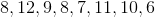In the set above, which is larger: the median, the mean, or the mode?

Mean

Mode

Median

Both median and mean

All are equal

Mean

Explanation:

Begin by ordering the set from smallest to largest: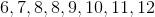Already, we see that the mode is 8. Find the median by taking the average of the two middle numbers: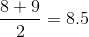Find the mean by adding all numbers and dividing by the total number of terms: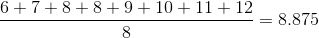Of the three, the mean of the set is the largest.

### Example Question #2 : How To Find Median

The grades on a test taken bystudents are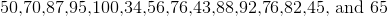respectively. What was the median score for this test?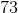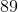Explanation:

To solve this problem, we must be aware of the definition of a median for a set of numbers. The median is defined as the number that is in middle of a set of numbers sorted from smallest to largest. Therefore we must first sort the numbers from largest to smallest.

34,43,45,50,56,65,70,76,76,82,87,88,92,95,100

43,45,50,56,65,70,76,76,81,87,88,82,95

45,50,56,65,70,76,76,81,87,88,82

50,56,65,70,76,76,81,87,88

56,65,70,76,76,81,87

65,70,76,76,81

70,76,76

76

Then by slowly eliminating the smallest and the largest numbers we find that the median score for this test is 76.

### Example Question #2 : Statistics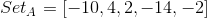Quantity A: The mean of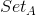Quantity B: The median ofThe relationship cannot be determined.

Quantity A is greater.

Quantity B is greater.

The two quantities are equal.

Quantity B is greater.

Explanation:

Begin by reordering the set in numerical order:Then becomes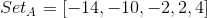Since there is an odd number of values, the median is the middle value.

Quantity B:Now, to find the arithmetic mean, take the sum of values divided by the total number of values.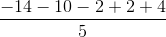Quantity A:### Example Question #1 : How To Find Median

The arithmetic mean of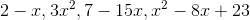is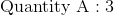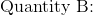The median of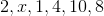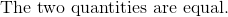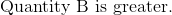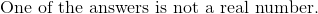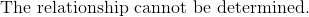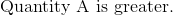Explanation:is an unknown value, but it can be found given what we know about the mean of the set: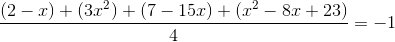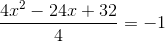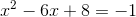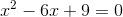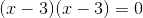Now,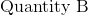is out of order; arrange in numerically: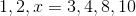Since there are even number of values, the median is the mean of the two middle most values: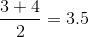### All GRE Math Resources